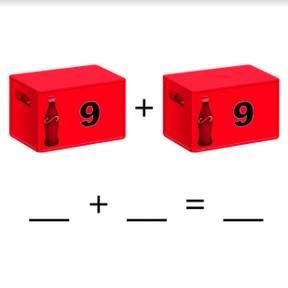Double and half math problems to 20

# Double and half math problems to 208,000 schools use Gynzy92,000 teachers use Gynzy1,600,000 students use Gynzy

## General

Students learn to solve double and half math problems with numbers to 20.

2.OA.B.2

## Relevance

When you are able to double or halve a number, you make solving math problems easier and quicker.

## Introduction

Ask students to count up to 20 by turns. Then they count back to 0 from 20 by turns. You may choose to challenge your students by asking them to skip count by twos when counting forward or backward.

## Development

Explain to students that doubling means adding the same number again. Show this using the dots. You can then use the red and white beads to show that you can double by counting the same amount again. Students first double with visual support and then are asked to double without visual support. Next explain that halving, or splitting in half, means splitting the number into two equal numbers. Show this using the dots. Then use the red and white beads to show halving into two equal groups of beads. Students first halve with visual support and then are asked to halve without visual support. Then explain to students that you can use the numbers from a problem in which you double to help solve a problem in which you halve. Show this with the colored numbers on the interactive whiteboard. Ask students to solve a few individually or in pairs.

To check that student are able to solve double and half math problems to 20, you can ask the following questions:
- What do you do when you double a number?
- What do you do when you halve a number?
- What is 8+8=? or 16 - 8 =?

## Guided practice

Students are first given problems in which they double or halve with visual support. Then they are asked to do so without visual support.

## Closing

Repeat the learning goal and why it is useful to be able to double or halve numbers. Next ask students to match the double and half math problems and solve them. Remind students that they can use the doubling math problem to solve the half math problem.

## Teaching tips

Students who have difficulties with this learning goal can make use of blocks or tokens to use while doubling and halving.

## Instruction materials

Optional: blocks or tokens

### The online teaching platform for interactive whiteboards and displays in schools

• Save time building lessons

• Manage the classroom more efficiently

• Increase student engagement